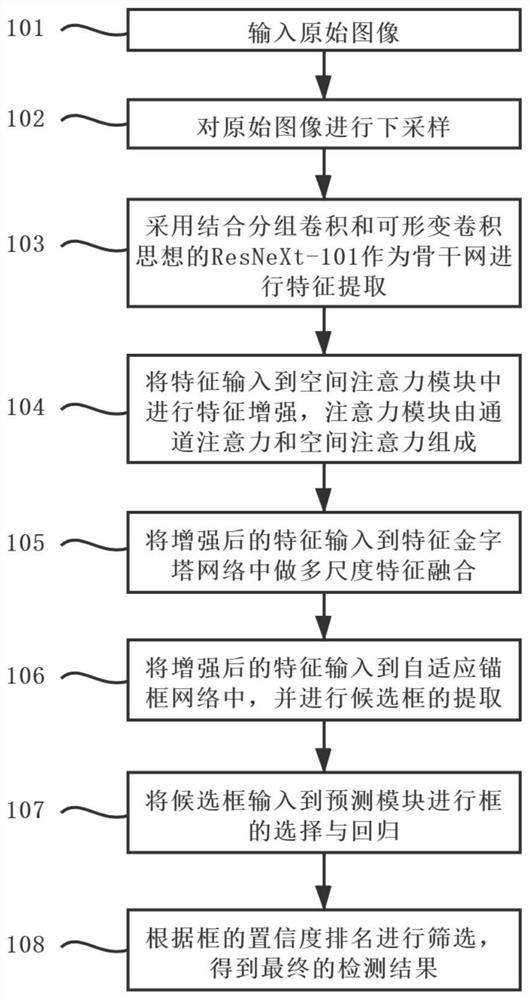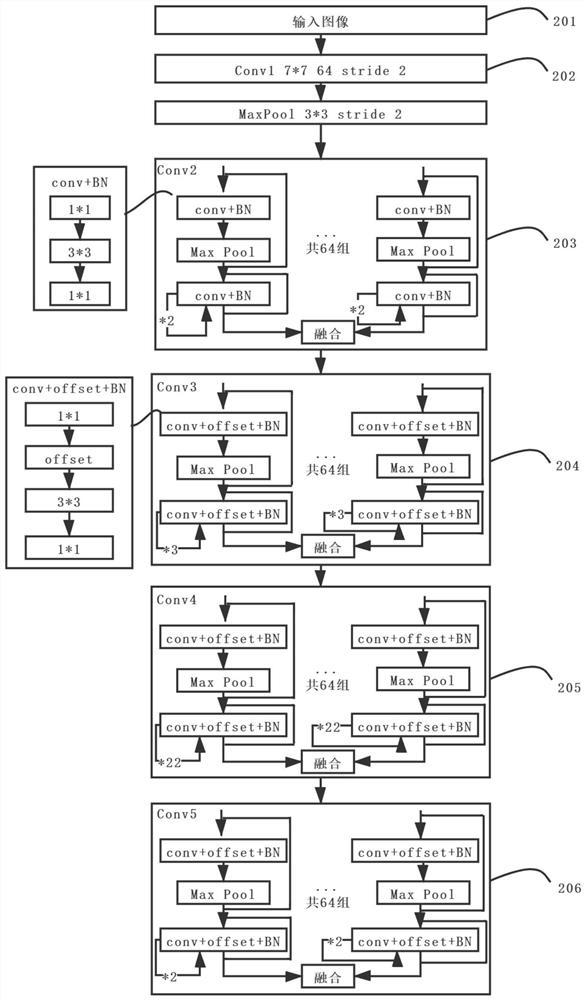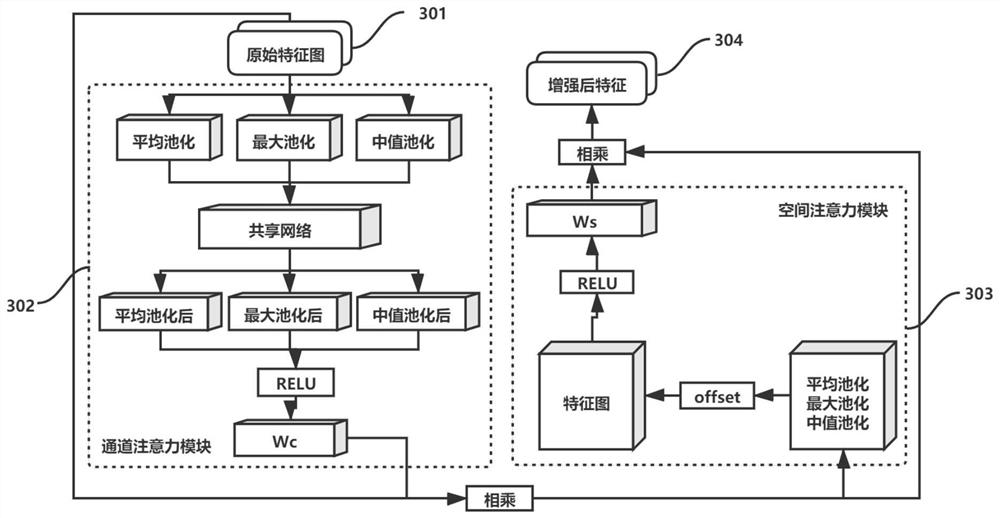# Aluminum material image defect detection method based on self-adaptive anchor frame

## A defect detection and self-adaptive technology, applied in the field of computer vision and defect detection, can solve the problems of inflexible detection methods and poor detection methods

Active Publication Date: 2020-12-15
XI AN JIAOTONG UNIV
8 Cites 19 Cited by

## AI-Extracted Technical Summary

### Problems solved by technology

 The purpose of the present invention is to provide an aluminum image defect detection method based on an adaptive anchor...
View more

### Method used

Step 106, step 105 result is input in adaptive anchor frame network, and carries out the extraction of candidate frame, this network can automatically select suitable anchor fr...
View more

## Abstract

The invention provides an aluminum material image defect detection method based on a self-adaptive anchor frame to solve the problems that a current defect detection method is not flexible enough, lowin detection precision and the like. Firstly, ResNeXt-101 using packet convolution and deformable convolution ideas is adopted as a backbone network, a feature enhancement module containing an attention mechanism is integrated into the backbone network, and then the feature enhancement module is sent into a feature pyramid network for multi-scale feature fusion, so that the defect detection precision is improved; secondly, a self-adaptive anchor frame neural network is used, corresponding anchor frame parameters are learned automatically according to defect features, and the precision of anchor frame positioning detection is improved; then, a cascade network structure is adopted in the frame prediction stage, and the problem that the precision in the training stage is not matched with that in the prediction stage is solved; and finally, the detection precision of the defects with large shape difference and small target defects is greatly improved, the overall precision of aluminum material image defect detection is relatively high, and the method has relatively high application value in the field of defect detection.

Application Domain

Image enhancementImage analysis +3

Technology Topic

## Image

•••## Examples

• Experimental program(1)

### Example Embodiment

 The invention will be further explained with reference to the following drawings:
 See Figure 1 The method comprises the following steps:
 Step 101: Use a camera to obtain image data or upload image data directly as image input.
 At step 102, the original image (W×S) is downsampled by s times to obtain an image of (W/s)×(H/s) size.
 Step 103: ResNeXt-101, which combines the idea of block convolution and deformable convolution, is used as the backbone network for feature extraction. After the original input image is processed by a convolution layer with a convolution kernel of 7×7 and a batch normalization layer, it is divided into 64 groups and entered into Conv2-Conv5. Block convolution can prevent over-fitting of a specific data set under the condition of constant parameters, thus achieving a more accurate effect.
 Step 104, input the features extracted in step 103 into the attention module for feature enhancement. The attention module consists of two sub-modules: the channel attention module and the spatial attention module. The structure diagram of this module is as shown in Figure 3. Figure 3.
 Step 105, input the feature map enhanced in step 104 into the feature pyramid network for multi-scale feature fusion, in which the top-level features are fused by upsampling and low-level features, but each layer will be independently predicted, so that the obtained features can represent defects more effectively.
 Step 106, input the result of step 105 into the adaptive anchor frame network, and extract the candidate frame. The network can automatically select the appropriate anchor frame according to the features, which reduces the error caused by manual setting and can better adapt to features of different sizes. The network structure is as follows Figure 4.
 Step 107, input the candidate box in step 106 into the prediction module to select and regress the candidate box, so as to find a more suitable candidate box.
 Step 108, screen according to that confidence rank of the candidate frames to obtain the final detection result.
 See Figure 2 Which depicts the structure diagram of the backbone network of the present invention, including the following parts:
Step 201: After preprocessing the pictures in the aluminum material defect data set, downsample them to the same size and input them into the backbone network.
 Step 202: After three groups of convolution layers with convolution kernel of 7×7 and step size of 2 and a batch normalization layer, the maximum pool operation of 3×3 is processed.
 In step 203, the backbone network adopts the idea of block convolution, and divides the output of step 202 into 64 groups. In each group, conv+BN consists of 1×1 convolution, 3×3 convolution group and 1×1 convolution. After 1×1 convolution, the feature map will be divided into different sub-feature maps according to the dimensions of the channel, in which the first sub-feature map will be directly output, and the rest will be output after 3×3 convolution. Each group adopts the idea of residual network, as follows Figure 2 Finally, these 64 groups of processed feature maps are fused.
 Step 204: Conv3 adds the idea of deformable convolution on the basis of Conv2, and adds an offset to each sampling point to help the network learn the features better. At the same time, it is divided into 64 groups to do grouping convolution operation. The details of the network are as follows Figure 2 Shown.
 Step 205: Conv4 adds the idea of deformable convolution on the basis of Conv2, and adds an offset to each sampling point to help the network learn the features better. At the same time, it is divided into 64 groups for grouping convolution operation. The details of the network are as follows Figure 2 Shown.
 Step 206: Conv5 adds the idea of deformable convolution on the basis of Conv2, adding an offset to each sampling point to help the network learn the features better, and at the same time, it is divided into 64 groups to do the grouping convolution operation, as shown in the network details. Figure 2 Shown.
 See Figure 3 Which depicts the structure diagram of the attention module of the present invention, including the following parts:
 Step 301, input the enhanced network feature map and output it to the channel attention module.
 Step 302, input that features output in step 301 into the channel attention module, which a c It is a one-dimensional channel attention feature map with a size of C×1×1, and its attention feature map A c (M(l, W, X)) is calculated as follows:

 Among them, Represents channel averaging pool operation, Represents the maximum channel pooling operation, Represents the pool operation of channel median, w 1 And W. 0 Is the weight learned by MLP, which can be used for all the input features, and RL stands for ReLU activation function, which is used to activate the feature vectors obtained by summing elements one by one.
 Step 303: Multiply the results obtained in step 302 and step 303 and send them to the spatial attention module, which a s It is a two-dimensional spatial attention characteristic map with a size of 1×W×H h. Spatial attention is the supplement of channel attention, which is described as:

 Among them, Stands for space average pooling operation, Stands for space maximum pooling operation, Mean value pooling operation in representative space, based on channel attention and input characteristics, through averaging pooling with deformable convolution. Maximal pooling And median pool. Get the spatial weight coefficient, RL represents ReLU activation function, γ. 7×7 Is a 7×7 convolution kernel with deformable convolution, and offset represents the offset.
 Step 304: Multiply the results obtained by the two attention modules to obtain the final feature, which means multiplying each element. M(l, W, X) represents the feature graph after the deformable convolution operation, which can be defined as:

 W(l n ) and w represent the learned weight, l n Represents any number in the real number set R, L is the parameter of linear interpolation, and X is the input characteristic map. B (,,) represents an N-dimensional bilinear interpolation algorithm, and its one-dimensional form is as follows:
 In which m i And n i Represents the case of m and n in the I dimension, respectively.
 M ′ (L, W, X) is the attention feature map formed by the relationship between channels, and M″(l, w″, X) is the final output feature map. Therefore, the formula of the deformation convolution module with attention mechanism is expressed as follows:
 M′(l，w′，x)＝A C (M(l，w，x))·M(l，w，x)，M″(l，w″，x)＝A s (M′(l，w′，x))·M′(l，w′，x)
 See Figure 4 Which depicts the structure diagram of the adaptive anchor frame network of the present invention, including the following parts:
 Step 401, input the feature map of the adaptive anchor frame network.
 At step 402, the input feature map is sent to the center coordinate prediction network, which is a binary classification network, and the network is based on the pixel points (I s ，j s ) generated a probability map P (| m I ), where s represents the relative distance of relevant anchor frames, m I Represents the characteristic map of an image, and the points on image I are generated by point-level convolution with activation functions. The network passes through the real coordinates of the central area (x g ，y g ) is mapped to the corresponding coordinates (x a ，y a ), marked as positive samples, and other coordinate points as negative samples, and then learn the generation model of the center point coordinates.
 Step 403, fuse the result obtained in step 402 with the input feature map to obtain a new feature map, and send it to the anchor frame length prediction network. The two networks of this network will predict the length of the anchor frame that is most suitable for each center point for each feature map, and get the mapping pH. Each network contains a pixel-level transformation layer with a size of 1×1×1, which is convenient for the subsequent steps to select candidate frames.
 Step 404, fuse the result obtained in step 402 with the input feature map to obtain a new feature map, and send it to the anchor frame width prediction network. These two networks will predict the width of the anchor frame most suitable for each center point for each feature map, and obtain the mapping pw. Each network contains a pixel-level transformation layer with a size of 1×1×1, which is convenient for the subsequent steps to select candidate frames.
Step 405: After step 403, many learned anchor frames will be generated for subsequent selection of suitable candidate frames.
 Step 406, that learn anchor frame is fused with the feature map by use a feature fusion network, and the merged features can adapt to the shape of the anchor frame at each position. The original feature map is corrected by 3×3 deformable convolution, and the offset is obtained by 1×1×2 convolution.
 Step 407: After the steps 405 and 406 are executed, the final feature map and candidate frame are obtained.
 See Figure 5 Which depicts a partial test result diagram of the present invention.
 The specific embodiments of the present invention have been described with reference to the attached drawings. Those skilled in the art should understand that the present invention is not limited by the above embodiments. On the basis of the technical scheme of the invention, various modifications or deformations that can be made by those skilled in the field without creative labor are still within the scope of protection of the invention.

## PUM## Description & Claims & Application Information

We can also present the details of the Description, Claims and Application information to help users get a comprehensive understanding of the technical details of the patent, such as background art, summary of invention, brief description of drawings, description of embodiments, and other original content. On the other hand, users can also determine the specific scope of protection of the technology through the list of claims; as well as understand the changes in the life cycle of the technology with the presentation of the patent timeline. Login to view more.
Who we serve
• R&D Engineer
• R&D Manager
• IP Professional
Why Eureka